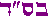D.A.F. >   Useful Resources > Emunah Issues > Torah Numerology[print version ]         [feedback ]
<<Previous: HomeThe Quintessential Number

What is the most perfect number in all of mathematics?

Without a doubt, it is none other than 2701 - the Gematria (numerical value) of Genesis 1:1.

You may ask, "well how do you measure perfection?"

Good question. Well, there is already a criteria! In math, there is an elite set of numbers called "perfect numbers". These are extremely rare. Only 51 are known (as of 2021). The fifth one is already greater than 33 million and the 16th has more than 1000 digits!

The criteria for "perfect numbers" is one simple symmetry. Namely, the number equals the sum of its divisors.

For example:
6 is a perfect number since it is divisible by 1,2,3 and 1+2+3=6.

Likewise, 28 is a perfect number since it is divisible by 1,2,4,7,14 and 1+2+4+7+14=28.

This simple symmetry was enough to classify it as a "perfect number".

Many of the ancient philosophers and theologians were awed by the 6 weekdays and the 28 day cycle of the moon. They saw this as a reflection of divine perfection in the world.
(note that the first word in Genesis 1:1 has 6 letters and the whole verse has 28 letters total. This is deep as we will see.)

Let's look at 2701.

This number has vastly more symmetry than any other number without comparison. In many ways, it is the supreme quintessential king of all numbers.

For example:
2701 = 37×73 (both prime factors are mirror reflections of each other)

Isn't that eye-catching?

2701 + 1072 (its own mirror reflection).
=3773 (same as the factors in order concatenated).

This double symmetry already makes it unique. No other known decimal number has this perfection.

How about the Geometric properties of 2701? Here too 2701 is replete with symmetries and perfection.

To name a few:
• 2701 is the 73rd triangle number - 1+2+3+4..+73 = 2701
• 2701 is the 37th hexagonal number
• both 37 and 73 are star (hexagram) numbers
• This makes 2701 a unique "star of stars" number as we'll see
It also has many unique and fascinating properties not shared by any other number. In short, it is the peerless numerical king of symmetry and perfection.

Let's have a closer look at Genesis 1:1

As part of the Oral Tradition from Sinai, every letter in the Hebrew language is assigned a numerical value. This is known as the Gematria.

 Gematria of Letters א ב ג ד ה ו ז ח ט י כ ל מ נ ס ע פ צ ק ר ש ת 1 2 3 4 5 6 7 8 9 10 20 30 40 50 60 70 80 90 100 200 300 400

Taking the Gematria of each letter in Genesis 1:1 and summing gives:
 Gematria of First Verse:"in the beginning G-d created the Heavens and the Earth" בְּרֵאשִׁ֖ית בָּרָ֣א אֱ-לֹהִ֑ים אֵ֥ת הַשָּׁמַ֖יִם וְאֵ֥ת הָאָֽרֶץ 913 203 86 401 395 407 296 Adding Gematrias of Words: 296+407+395+401+86+203+913 Total=2701

Thus it seems the totality of the verse has been tuned to 2701.

 Gematria of First Verse בְּרֵאשִׁ֖ית בָּרָ֣א אֱ-לֹהִ֑ים אֵ֥ת הַשָּׁמַ֖יִם וְאֵ֥ת הָאָֽרֶץ 913 203 86 401 395 407 296 Multiplying Gematrias of Words: 296×407×395×401×86×203×913 =304,153,525,784,175,760 Sum of the Thousands: 304+153+525+784+175+760 Total=2701Incredible! Not only the total verse is tuned to 2701 but also the balance of words is finely tuned to 2701. (note: the "sum of thousands" is how large numbers are represented in Hebrew due to lack of zero)

With this introduction, let us begin the descent into the bottomless depths of divine wisdom. Please hold on to the railing.

>> Next: Wisdom = Yud

Footnotes
•  this summing of groups of 3 is known as "sum of thousands". In Hebrew there is no zero so large numbers are grouped into thousands. For example, the Hebrew date is 5780. One could write it out fully with 14 Tavs, etc. But instead a short-hand method is used by grouping the thousands. So 5 thousand becomes just "Heh" (5) and Tav-Shin-Peh (780). The "sum of thousands" is the sum of these groups. The lack of zero is due to the Hebrew letters having a reality in higher realms. return to text

Mirrored from dafyomireview.com/543
contact